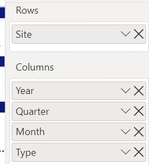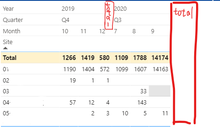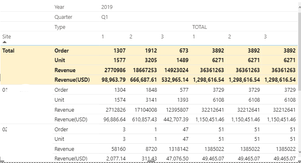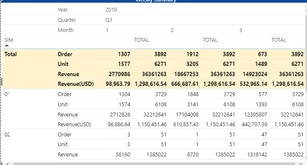cancel
Showing results for
Did you mean:Frequent Visitor

## Total column layout unexpected

Hi,guys!
I'm a new for PowerBI,having a solution,almost 3 days thinking with it,and that make me crazy.
Please give me some suggestion with below:

A matrix,
row:  shop_item
column: Year、Quartar、Month、typevalue: Order、Unit、Revenue (All are the measure with sum)

I want to show data  in the quarter which the user select,and need to compare another quarter the user also selected.
so,I have two Calendar filter like thisand my matrix like thisBecause I need to compare with two quarters,I need the total value with 3 months.
I have tried many ways to put the total column like thiscolumn subtotal is no work,and i use a custom table to switch the value,the layout will be change like thisthe value measure code like this

``````Test thisQMaxMonth_Order1 =
var _thisQMaxMonth = MAX(DIM_Calendar[Month])
VAR _YQ0 = SELECTEDVALUE('DIM_Calendar'[Year])*100 + (4-SELECTEDVALUE('DIM_Calendar'[QuarterOrder]))
VAR _YQ1 = SELECTEDVALUE('DIM_Calendar (2)'[Year])*100 + (4-SELECTEDVALUE('DIM_Calendar (2)'[QuarterOrder]))
VAR _VALUE1 = CALCULATE([#Order],FILTER('DIM_Calendar (2)'
,'DIM_Calendar (2)'[Month] = _thisQMaxMonth)
,USERELATIONSHIP('DIM_Calendar (2)'[Date], 'FACT_Sales'[Order_Creation_Date]), ALL('DIM_Calendar'))
var _totalvalue1 = CALCULATE([#Order]
,USERELATIONSHIP('DIM_Calendar (2)'[Date], 'FACT_Sales'[Order_Creation_Date]), ALL('DIM_Calendar'))

return
SWITCH( SELECTEDVALUE('Type'[Type])
,"", SWITCH(_YQ0, _YQ1, _VALUE1)
,"total", SWITCH(_YQ0, _YQ1, _totalvalue1)
)``````

Finally,I try to create a table including month(1-12 + total ) or Quarter(Q1-Q4 + total),but still no work...
I really no ideal How to do this in one matrix!
Could someone give me a suggestion,i will appreciate that.Community Support

Hi  @JosephWu ,

Could you pls share your pbix file ,remember to remove confidential data.

Best Regards

Lucien